# ttplot

Plot threshold transitions

## Syntax

``ttplot(tt)``
``ttplot(tt,Name,Value)``
``ttplot(ax,___)``
``h = ttplot(___)``

## Description

`ttplot` plots transition functions of threshold transitions. To evaluate the transition function for observations of the threshold variable, use `ttdata`.

example

````ttplot(tt)` plots transition bands between states of the threshold transitions `tt` on the y-axis. The plot shows gradient shading of the mixing level in the transition bands.```

example

````ttplot(tt,Name,Value)` uses additional options specified by one or more name-value arguments. For example, `ttplot(tt,Type="graph")` specifies plotting a line plot of the transition function at each threshold level on the same axes.```
````ttplot(ax,___)` plots on the axes specified by `ax` instead of the current axes (`gca`) using any of the input argument combinations in the previous syntaxes.```
````h = ttplot(___)` returns a handle `h` to the threshold transitions plot. Use `h` to modify properties of the plot after you create it.```

## Examples

collapse all

Create discrete threshold transitions at 0 and 2.

```t = [0 2]; tt = threshold(t)```
```tt = threshold with properties: Type: 'discrete' Levels: [0 2] Rates: [] StateNames: ["1" "2" "3"] NumStates: 3 ```

`tt` is a `threshold` object. The specified thresholds split the space into three states.

Plot the threshold transitions.

`ttplot(tt);`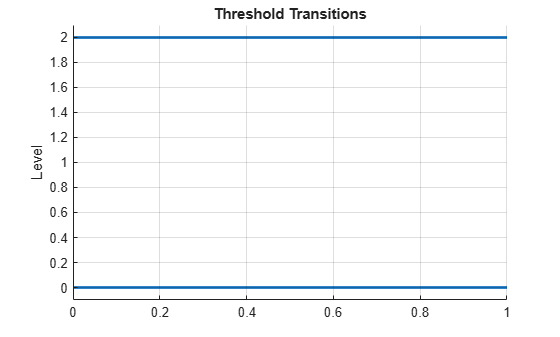`ttplot` graphs a gradient plot by default. The $\mathit{y}$-axis represents the value of the threshold variable ${\mathit{z}}_{\mathit{t}}$ (currently undefined) and the state-space:

• The system is in state 1 when ${\mathit{z}}_{\mathit{t}}<0$.

• The system is in state 2 when $0\le {\mathit{z}}_{\mathit{t}}<2$.

• The system is in state 3 when ${\mathit{z}}_{\mathit{t}}\ge 2$.

Because the transitions are discrete, `ttplot` graphs the levels as lines—the regime switches abruptly when ${\mathit{z}}_{\mathit{t}}$ crosses a threshold variable.

Because ${\mathit{z}}_{\mathit{t}}$ is undefined, the $\mathit{x}$-axis is arbitrary. When you specify threshold variable data by using the `Data` name-value argument, the $\mathit{x}$-axis is the sample index.

This example shows how to create two logistic threshold transitions with different transition rates, and then display a gradient plot of the transitions.

Load the yearly Canadian inflation and interest rates data set. Extract the inflation rate based on consumer price index (`INF_C`) from the table, and plot the series.

```load Data_Canada INF_C = DataTable.INF_C; plot(dates,INF_C); axis tight```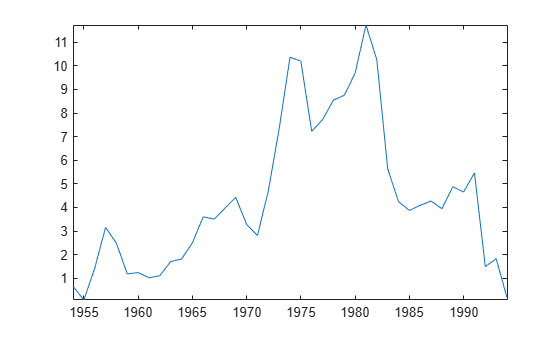Assume the following characteristics of the inflation rate series:

• Rates below 2% are low.

• Rates at least 2% and below 8% are medium.

• Rates at least 8% are high.

• A logistic transition function describes the transition between states well.

• Transition between low and medium rates are faster than transitions between medium and high.

Create threshold transitions to describe the Canadian inflation rates.

```t = [2 8]; % Thresholds r = [3.5 1.5]; % Transition rates statenames = ["Low" "Med" "High"]; tt = threshold(t,Type="logistic",Rates=r,StateNames=statenames)```
```tt = threshold with properties: Type: 'logistic' Levels: [2 8] Rates: [3.5000 1.5000] StateNames: ["Low" "Med" "High"] NumStates: 3 ```

Plot the threshold transitions; show the gradient of the transition function between the states, and overlay the data.

```figure ttplot(tt,Data=INF_C)```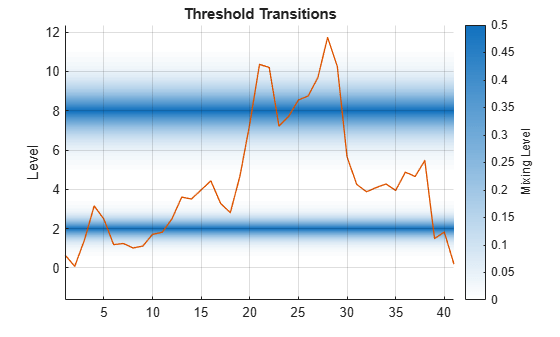Create normal cdf threshold transitions at levels 0 and 5, with rates 0.5 and 1.5.

```t = [0 5]; r = [0.5 1.5]; tt = threshold(t,Type="normal",Rates=r)```
```tt = threshold with properties: Type: 'normal' Levels: [0 5] Rates: [0.5000 1.5000] StateNames: ["1" "2" "3"] NumStates: 3 ```

To compare the behavior of the transition functions, plot their graphs at the same level.

```figure ttplot(tt,Type="graph",Width=20)```Plot the transition functions at their levels. Evaluate the transition function over a 1-D grid of values by using `ttdata`, and then plot the results.

```lower = tt.Levels(1) - 3/min(tt.Rates); upper = tt.Levels(end) + 3/min(tt.Rates); z = lower:0.1:upper; F = ttdata(tt,z,UseZeroLevels=false); figure plot(z,F,LineWidth=2) grid on xlabel("Level") legend(["Level 0, Rate 0.5" "Level 5, Rate 1.5"],Location="NorthWest")```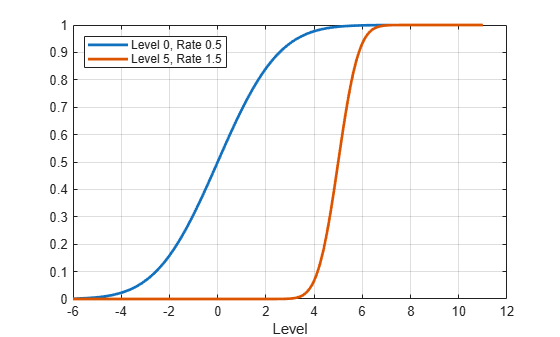Create smooth threshold transitions for the Australian to US dollar exchange rate to model price parity.

Load the Australia/US purchasing power and interest rates data set. Extract the log of the exchange rate `EXCH` from the table.

```load Data_JAustralian EXCH = DataTable.EXCH; ```

Consider a two-state system where:

• State 1 occurs when the Australian dollar buys more than the US dollar (`EXCH` $\ge 0$).

• State 2 occurs when the US dollar buys more than the Australian dollar (`EXCH` $<\mathrm{0}$).

• States are weighed more highly as the system deviates from parity (`EXCH` = 0).

Create threshold transitions representing the system. To attribute a greater amount of mixing away from the threshold, specify an exponential transition function. Set the transition rate to 2.5.

`tt = threshold(0,Type="exponential",Rates=2.5)`
```tt = threshold with properties: Type: 'exponential' Levels: 0 Rates: 2.5000 StateNames: ["1" "2"] NumStates: 2 ```

Plot the threshold transitions with the threshold data.

```figure ttplot(tt,Data=EXCH);```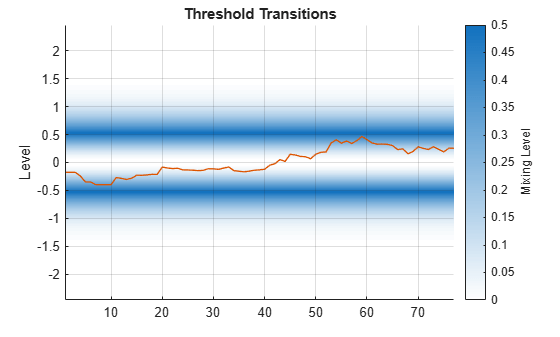Try improving the display by experimenting with the transition band width (`Width` name-value argument).

```figure ttplot(tt,Data=EXCH,Width=2);```Plot the transition function.

```figure ttplot(tt,Type="graph");```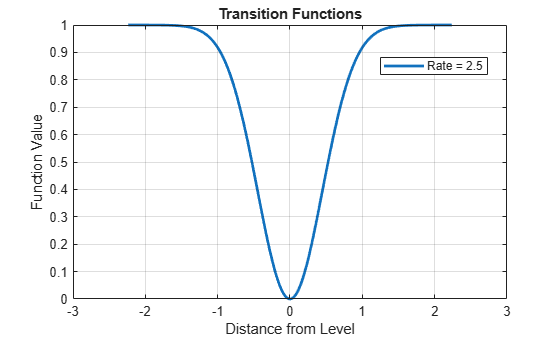## Input Arguments

collapse all

Threshold transitions, with `NumStates` states, specified as a `threshold` object. `tt` must be fully specified (no `NaN` entries).

Axes on which to plot, specified as an `Axes` object.

By default, `ttplot` plots to the current axes (`gca`).

### Name-Value Arguments

Specify optional pairs of arguments as `Name1=Value1,...,NameN=ValueN`, where `Name` is the argument name and `Value` is the corresponding value. Name-value arguments must appear after other arguments, but the order of the pairs does not matter.

Before R2021a, use commas to separate each name and value, and enclose `Name` in quotes.

Example: `Type="graph"` specifies plotting a line plot of the transition function at each threshold level on the same axes.

Plot type, specified as a value in this table.

ValueDescription
`"gradient"`Gradient shading of the mixing level in each transition band.
`"graph"`

Graphs of transition functions at each level.

`ttplot` plots graphs with levels set to zero.

Example: `Type="graph"`

Data Types: `char` | `string`

Data on a threshold variable zt to include in the plot, specified as a numeric vector.

`ttplot` plots `Data` with gradient shading of transition bands (`Type="gradient"`). If `Type="graph"`, `ttplot` ignores `Data`.

Data Types: `double`

Width of transition bands, specified as a positive numeric scalar.

• For gradient plots (`Type="gradient"`), `ttplot` truncates transition function data outside of the bands.

• For transition function graphs (`Type="graph"`), `ttplot` sets the x-axis limits to `[-Width/2 Width/2]`.

By default, `ttplot` selects the band width automatically.

Example: `Width=10`

Data Types: `double`

## Output Arguments

collapse all

Plot handle, returned as a graphics object. `h` contains a unique plot identifier, which you can use to query or modify properties of the plot.

collapse all

### Mixing Level

The mixing level is the degree to which adjacent states contribute to a response.

Transition functions F vary between 0 and 1; adjacent states are assigned weights F and 1 – F. The mixing level between adjacent states is the minimum weight min(F, 1 – F).

The following characteristics define the mixing behavior of each transition type:

• Discrete transitions have no mixing.

• Normal and logistic transitions achieve maximum mixing at threshold levels.

• Exponential transitions achieve maximum mixing on either side of threshold levels.

For more details, see `threshold`.

## Tips

• Use the `Width` name-value argument to adjust the display of transition function graph (`Type="graph"`) plots with varying rates. In multilevel gradient plots (`Type="gradient"`), a large enough width results in overlapping transition bands that can misrepresent data. By default, `ttplot` chooses an appropriate width for displaying all transitions.

## Version History

Introduced in R2021b